# Electrical Characteristics of Diodes

## Static Characteristics

The following figure shows the static characteristic of diodes.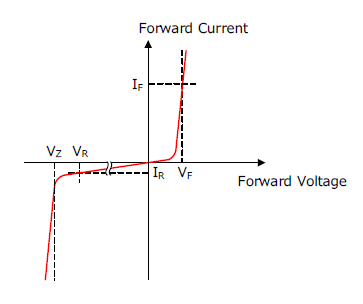### Forward voltage drop, VF, forward current, IF

The current that flows when a voltage is applied in the forward direction is called the forward current, IF. The voltage when IF flows is called the forward voltage drop, VF.
When comparing the IF – VF characteristics of diodes, the lower the VF required to flow the same amount of IF, the lower the power loss and the better the characteristics.
VF has a negative temperature characteristic and thus the higher the temperature, the lower the VF.

### Reverse voltage, VR, reverse leakage current, IR

The current that flows when a voltage is applied in the reverse direction is called the reverse leakage current, IR. The voltage when IR flows is called the reverse voltage, VR.
When a voltage is applied in the reverse direction, a slight leakage current, IR, flows. A diode with a smaller IR has less power dissipation and can prevent thermal runaway.
IR has positive temperature characteristics, and thus the higher the temperature, the higher the IR.

### Breakdown Voltage, VZ

When the reverse voltage, VR, is increased, the reverse leakage current, IR, increases sharply at a certain voltage. This voltage is called the breakdown voltage, VZ. The breakdown voltage is also called the Zener voltage.

## Switching Characteristic

As shown in the following figure, the recovery current flows when the reverse voltage is applied from the state where the forward voltage is applied by turning the switch. The time from when the recovery current flows until the recovery current decreases is called the Reverse Recovery Time, trr. The larger the forward current, IF, the longer the trr. Since the recovery current causes noise and power loss, the shorter the trr, the better the characteristics.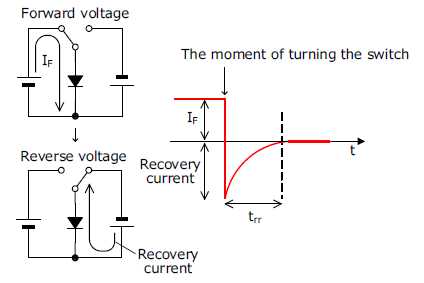The following describes the reason why the recovery current flows when a voltage changes from the forward voltage to the reverse voltage.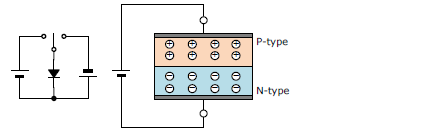A: When no voltage appliedHoles and electrons are in equilibrium.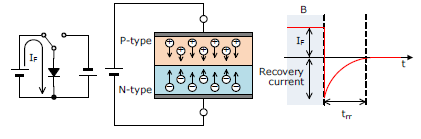B: When forward voltage appliedElectrons move to P-type semiconductors and holes move to N-type semiconductors (i.e., IF is flowing).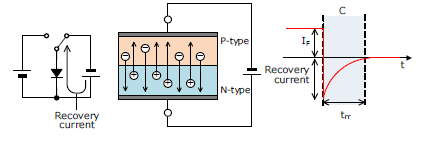C: The moment when the reverse voltage is appliedAt the moment the switch is turned, a reverse voltage is applied to the diode, and the directions of movement of electrons and holes are reversed. The current that flows at this time is the recovery current.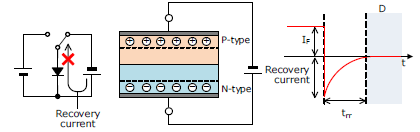D: When reverse voltage appliedAfter a moment, the depletion layer expands and holes and electrons do not move. The time from C to D is trr.